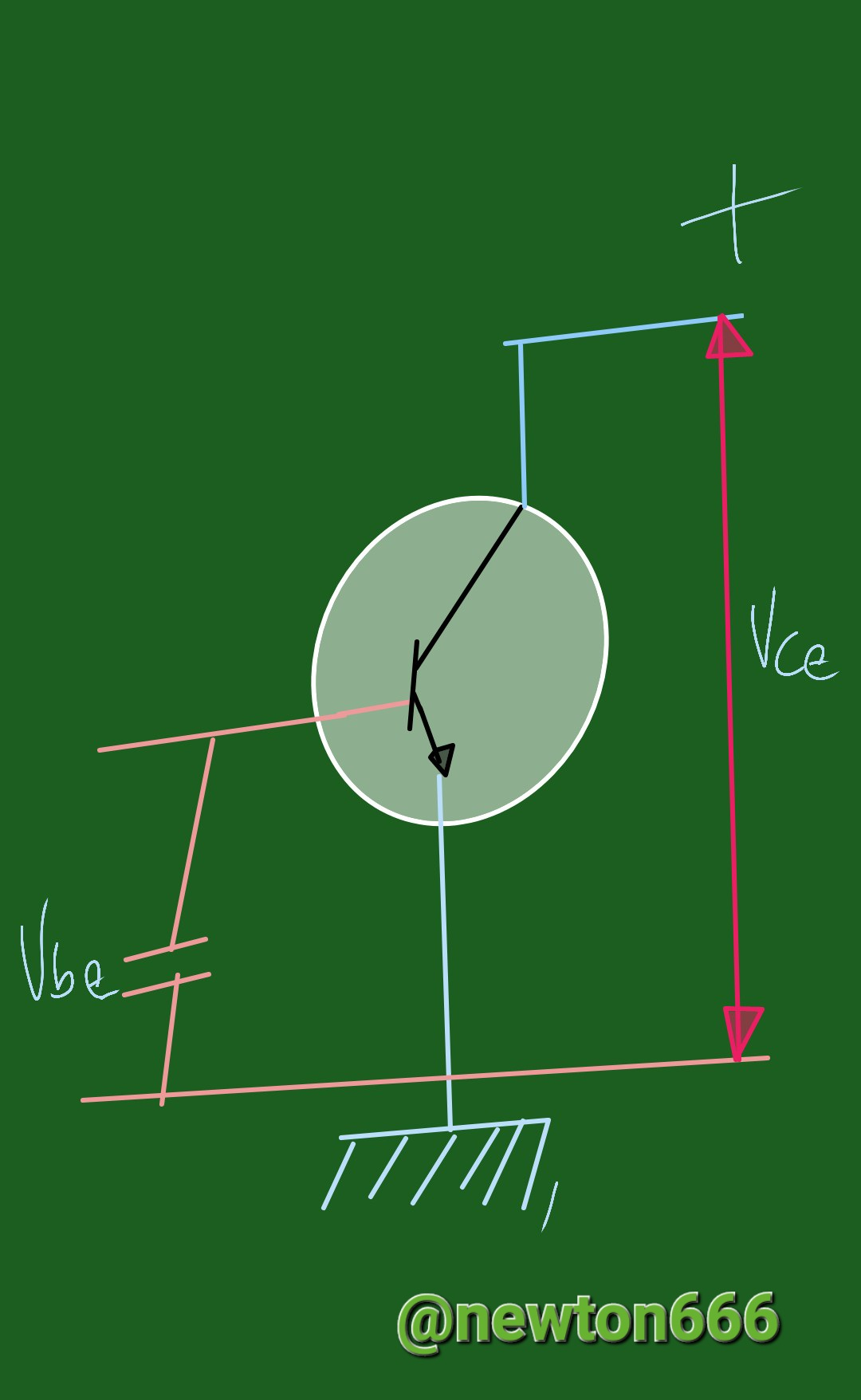# Voltage and current in the transistor of a circuit

in STEMGeeks3 months ago
Hello greetings to all science lovers, this time I bring you the level of circuit physics, ransistors are semiconductor devices that regulate the movement of electric current in electrical circuits. The base, collector, and emitter are the three terminals on a transistor. The operation of the transistor and, by extension, the circuit in which it is installed depend on the current and voltage at each of these terminals.We have the following representation where my reader friends, we have the polarization in a circuit, which a transistor performs the function of passing current to a collector collector, by means of a positive charge power, also connected to a battery at the base of the circuit, the singularity of all this is reinforced by resistance, where the force of the potential is reflected in the collector and the emitter.

The movement of electrons via a transistor is referred to as current. Both the base and the collector carry current, which then travels to the emitter. The transistor's base current regulates the current that travels from the collector to the emitter. This is so that the transistor's resistance, which in turn regulates the transistor's current in the base, can alter.

The difference in electrical potential between the transistor's terminals is referred to as voltage. The current that travels from the collector to the emitter is determined by the voltage at the base. The transistor is said to be in active mode when the voltage at the base is greater than the voltage at the emitter. The transistor functions as a switch in this mode, allowing a sizable current to travel from the collector to the emitter. The transistor is said to be in cutoff mode when the voltage at the base is lower than the voltage at the emitter.
When operating in this state, the transistor behaves like an open switch and prevents current from the collector from flowing.

The current gain equation, which illustrates the relationship between the current running through the collector and the current flowing through the base, is an illustration of an equation relevant to transistor operation.

The transistor's collector current (IC) to base current (IB) ratio is known as the current gain (hFE):
hFE = IC / IB

The voltage divider rule, which is used to determine the voltage at the transistor's base in a circuit, is another significant equation associated with transistor operation. According to the voltage divider rule, the voltage at the base (VB) equals the product of the circuit's overall voltage (VT) and the ratio of the resistance between the base and emitter (RB) to the circuit's overall resistance (RT)
VB = VT * (RB / RT)

For example, consider a circuit with a total voltage of 12 volts, a total resistance of 1000 ohms, and a base-emitter resistance of 100 ohms. Using the voltage divider rule, we can calculate the voltage at the base of the transistor as:

VB = 12 * (100 / 1000) = 1.2 volts

Depending on the voltage at the emitter and the properties of the transistor, this voltage can then be used to identify whether the transistor is operating in active mode or cutoff mode.

Photo edited by my Samsung A23 phone

Bibliography Reference

Electronics Practices by Paul B. Zbar, ‎Albert Paul Malvino, ‎Michael A. Miller, 2003.

Physics for science and technology. II by Paul Allen Tipler, ‎Gene Mosca, 2004.Posted with STEMGeeks

Sort:
3 months ago Reveal Comment